*项目中的模型来自 R Gem Effect Unity Plugin ，HDR 环境图来自 HDRIHaven

##### 实时渲染宝石

1. 整个场景使用光栅化渲染流程，只有在渲染宝石物体时，使用光线追踪，在片元着色器中从摄像机放射光线。
2. 每个宝石物体在光线追踪时，只和自己的 Mesh 模型进行求交点的计算。
3. 只使用光线追踪来计算光线的折射和反射。
4. 假设宝石表面是完全光滑的，不考虑微表面，且内部无任何杂质。其表面只有 specular 项，无 diffuse 项。
5. 光线仅在第一次与宝石表面相交时，被分为反射光线和折射光线，之后折射光线在宝石的内部进行全反射或其折射出宝石表面。
6. 光线经过反射或折射，射出宝石表面后，对天空球进行采样。

##### 折射

float Refract(float3 i, float3 n, float eta, inout float3 o)
{
float cosi = dot(-i, n);
float cost2 = 1.0f - eta * eta * (1 - cosi * cosi);

o = eta * i + ((eta * cosi - sqrt(cost2)) * n);
return 1 - step(cost2, 0);
}


float eta;
float3 normal;

// out
if (dot(ray.direction, hit.normal) > 0)
{
normal = -hit.normal;
eta = _IOR;
}
// in
else
{
normal = hit.normal;
eta = 1.0 / _IOR;
}

ray.origin = hit.position - normal * 0.001f;

float3 refractRay;
float refracted = Refract(ray.direction, normal, eta, refractRay);

……

// Refraction
if (refracted == 1.0)
ray.direction = refractRay;
// Total Internal Reflection
else
ray.direction = reflect(ray.direction, normal);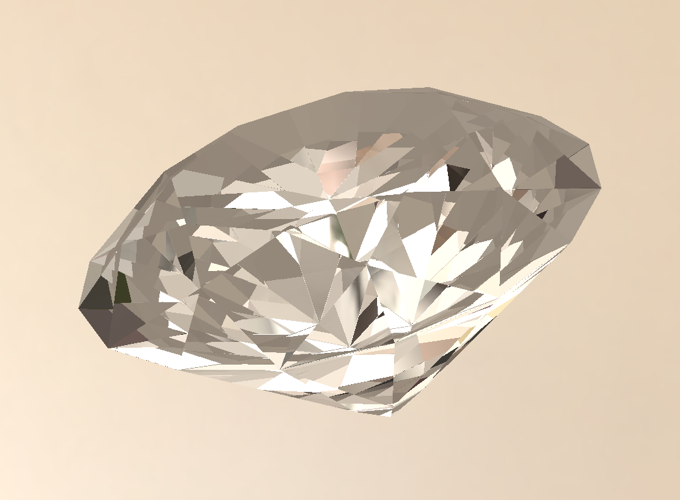##### 反射

float3 reflect(float3 i, float3 n)
{
return i - 2.0 * n * dot(n, i);
}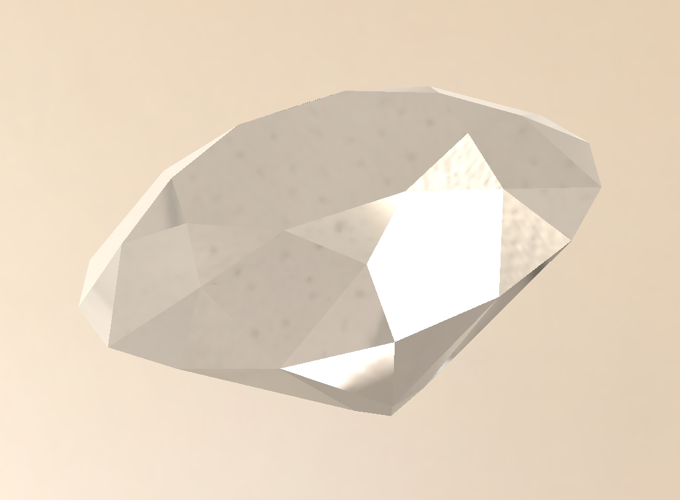##### 菲涅尔

float FresnelSchlick(float3 normal, float3 incident, float ref_idx)
{
float cosine = dot(-incident, normal);
float r0 = (1 - ref_idx) / (1 + ref_idx); // ref_idx = n2/n1
r0 = r0 * r0;
return r0 + (1 - r0) * pow((1 - cosine), 5);
}


if (depth == 0)
{
float3 reflectDir = reflect(ray.direction, hit.normal);
reflectDir = normalize(reflectDir);

float3 reflectProb = FresnelSchlick(normal, ray.direction, eta) * _Specular;
specular = SampleCubemap(reflectDir) * reflectProb;
ray.energy *= 1 - reflectProb;
}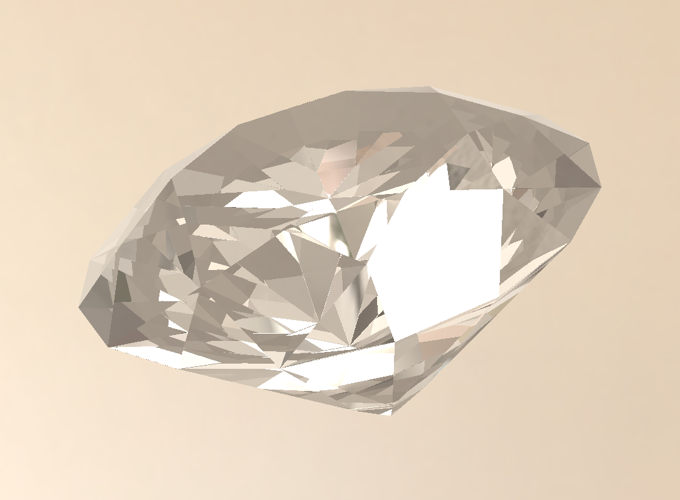##### 光线吸收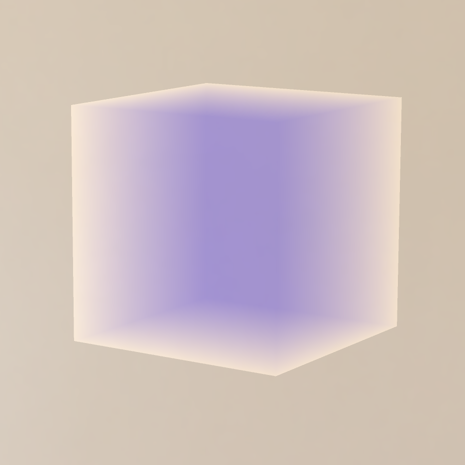struct Ray
{
float3 origin;
float3 direction;
float3 energy;
float absorbDistance;
};


Shade 函数中对累加计算：

float3 Shade(inout Ray ray, RayHit hit, int depth)
{
if (hit.distance < 1.#INF && depth < (_TraceCount - 1))
{
……
if (depth != 0)
ray.absorbDistance += hit.distance;
……
}
}


float3 Shade(inout Ray ray, RayHit hit, int depth)
{
if (hit.distance < 1.#INF && depth < (_TraceCount - 1))
{
……
}
else
{
ray.energy = 0.0f;

float3 cubeColor = SampleCubemap(ray.direction);
float3 absorbColor = float3(1.0, 1.0, 1.0) - _Color;
float3 absorb = exp(-absorbColor * ray.absorbDistance * _AbsorbIntensity);

return cubeColor * absorb * _ColorMultiply + _Color * _ColorAdd;
}
}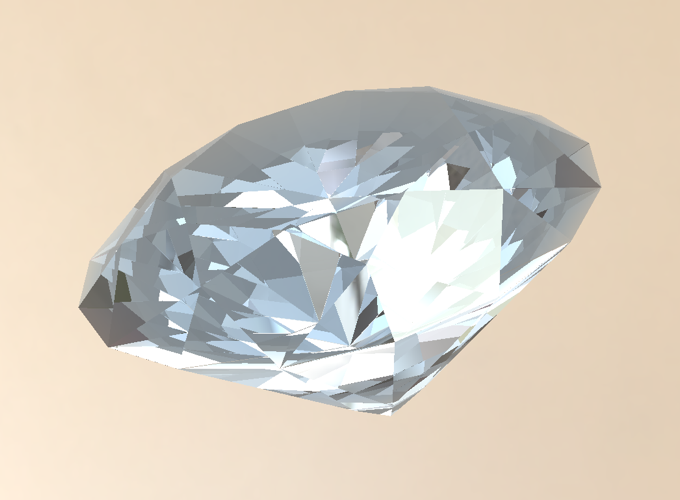##### 最终效果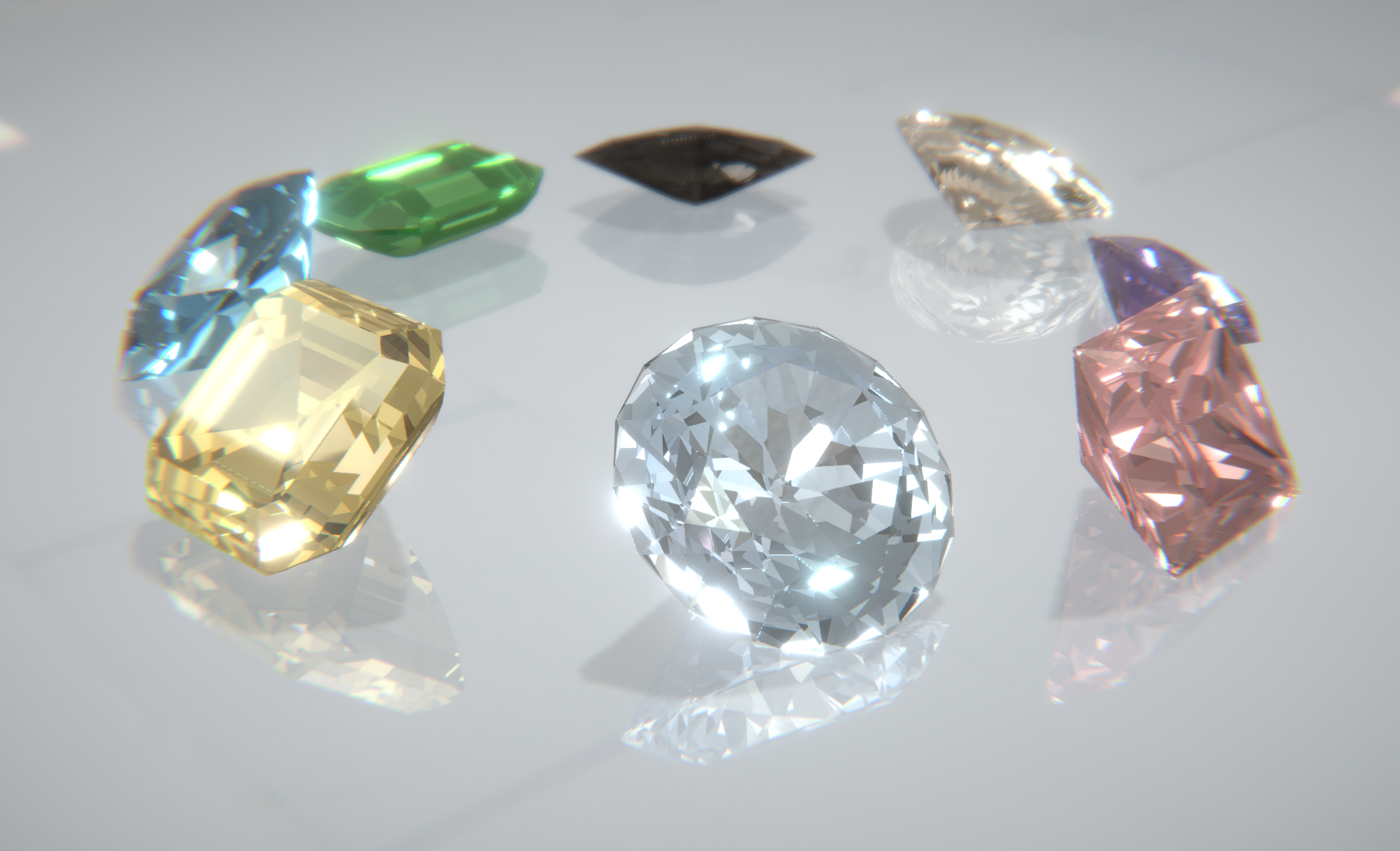##### 扩展阅读

“Cheap” Diamond Rendering

##### 参考

GPU Ray Tracing in Unity

Ray Tracing in One Weekend

Triangle Intersection

Reflection, Refraction and Fresnel

Raytracing Reflection, Refraction, Fresnel, Total Internal Reflection, and Beer’s Law

Microfacet models for refraction through rough surfaces.

Extending the Disney BRDF to a BSDF with Integrated Subsurface Scattering